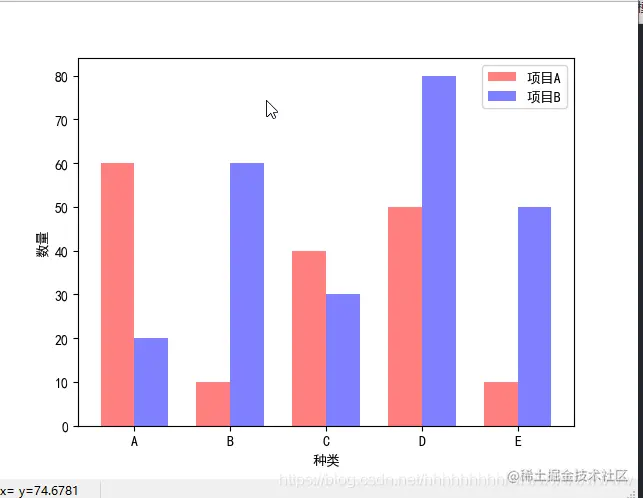# Python pyplot draws a parallel histogram, and the x-axis value is displayed in the center of the two histograms

2022-01-30 08:54:53 AI Hao

``````import matplotlib.pyplot as plt
import matplotlib as mpl
import numpy as np

mpl.rcParams["font.sans-serif"] = ["SimHei"]
mpl.rcParams["axes.unicode_minus"] = False

x = np.arange(5)
y = [60, 10, 40, 50, 10]
y1 = [20, 60, 30, 80, 50]

bar_width = 0.35
tick_label = ["A", "B", "C", "D", "E"]

plt.bar(x, y, bar_width, align="center", color="red", label=" project A", alpha=0.5)
plt.bar(x+bar_width, y1, bar_width, color="blue", align="center", label=" project B", alpha=0.5)

plt.xlabel(" species ")
plt.ylabel(" Number ")

plt.xticks(x+bar_width/2, tick_label)

plt.legend()

plt.show()
Copy code ``````